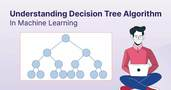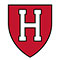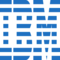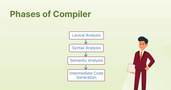Basics of Machine Learning – Definition and Concepts

# Basics of Machine Learning – Definition and Concepts

clickHere
Updated on Oct 27, 2023 17:36 IST

This post will help you understand the emerging technology of today’s time- Machine Learning. Here we have covered basic concept of Machine Learning.

Machine learning has been a hot topic in technology discussions for some time. Every organization is trying to leverage its power. So, what is Machine Learning?

## Definition of Machine Learning

We can define machine learning by listing its key features below;

• It uses mathematical models to make inferences from the example data
• Builds the mathematical models using example data/past experience

Tom Mitchell, a famed Professor at Carnegie Mellon University defines Machine Learning as follows:

“A computer program is said to learn from experience E with respect to some class of tasks T and performance measure P, if its performance at tasks in T, as measured by P, improves with experience E. “

In fig.1 below, we can see that in machine learning, the model learns the formula/equation from the data (set of input and corresponding outputs) as opposed to usual computations, where we know the formula/equation and get the output by supplying the input to the formula.

Fig 1: In machine learning, the model learns the formula based on the patterns in the input data

As we know, machine learning is used in many applications today. In fact, almost every AI application is powered by Machine Learning algorithms. Notably, it is used in applications such as web search, e-mail spam detection, credit card fraud detection, product recommendations, medical diagnosis, predictive maintenance, etc.

Must Explore – Machine Learning Courses

## The Jargon of Machine Learning

Before we move further, we should understand jargon related to Machine Learning.

·     Features-Distinct traits used to describe each item in a quantitative manner. For example – house price (Y) depends on many input features (X1, X2, …Xn) such as location, no. of bedrooms, park facing or not, age of the property, plinth area, etc.

·     Bias- How much does the average model overall training sets differ from the true model? This is the error due to inaccurate assumptions/simplifications made by the model. Bias would be high when we omit some important independent variables (Xi’s) over which Y depends

·     Variance- How much models estimated from different training sets differ from each other. The variance would be high if we try to build our model suited to one specific training set (called overfitting). The model then would show less error for that training set but would not generalize well i.e. would show high error for test data.

·     Training data – The input data is divided into training and test data. The model is built/learned with this training data.

·     Validation data – Part of the training data is used to validate the model before testing it on unseen data. Validation is like providing the model with mock tests before the actual test. Please note that the validation data is not used for training purposes. It is used to validate the already-trained model

·     Test data – Test data is used to test the model’s accuracy. If the model demonstrates high enough accuracy with test data, it would be accepted for deployment

·     Labeled data – Data consisting of a set of training examples, where each example is a pair consisting of an input and the desired output value i.e. sets of (X, Y) where for every ‘X’, there is a corresponding ‘Y’.

·     Unlabeled Data – Data where there is no ‘Y’ corresponding to ‘X’. The learning here consists of identifying the pattern within data and classifying it into various categories/clusters

·     Categorical Variable – In input features for labelled data, there might be some variables that have non-numerical values such as ‘Yes’, No’, or some other category. These categorical variables are then assigned some numerical values for further processing – e.g. assigning 1 to ‘Yes’, and 0 to ‘No’.

## Types of Machine Learning Algorithms:

There are many algorithms for machine learning. It’s an ever-evolving field with new developments happening at leading research universities across the world and also in leading companies like Google’s DeepMind, Google Brain, Open AI Foundation, Tesla and Facebook, etc. Primarily, the machine learning algorithm could be clubbed under the following three broad categories.

A)  Supervised Learning

B)  Unsupervised Learning

C)  Reinforcement Learning

Let’s understand what each type of learning entails:

A)  Supervised Learning: This learning works with labelled data. A set of X, and Y (pre-classified training examples) are given. The model is trained on this data and then for a new observation (input) X, the model tells us the predicted value of Y.

Some of the algorithms used for supervised learning are as follows;

·     Regression

·     Decision Trees

·     K Nearest Neighbor

·     Naive Bayes

·     Support Vector Machines (SVM)

·     Neural Networks

Fig 2: Supervised Learning works with the labelled data and learns the model

Broadly, the supervised learning problems could be further divided into two categories

i.     Regression – Given a set of input features X1, X2, …, Xn, a target feature Y is predicted. Y is a continuous function. Examples of regression are predicting housing prices, predicting sales volume, predicting market demand, etc. Regression could be linear or nonlinear. For example,

y=w”0″+w1*x1+w2*x2+⋯.+wn*xn

is an example of linear regression. Here  x1, x2 ..xn are input features affecting house price. y is house price and w0, w1, w2, …wn are the parameters of the model. Similarly,

y=w0+w1*x12+w2*x22+……..+ wn* xn2​

is an example of non-linear regression. Equation of Y with any power of Xis or with some exponential or logarithmic or trigonometric function of Xi would be non-linear

ii. Classification – Same as regression except here the target feature Y is discrete. i.e. It could either be binary or multiclass. An example of binary Y (output) is ‘Yes’, or ‘No’ (as in predicting whether someone would be Covid positive given his weight, age, co-morbidity, etc.). An example of multiclass Y is predicting the grade of a student (out of A,B,C,D,F), predicting the type of weather (sunny, rainy, cloudy with no rain, part sunny and part cloudy), etc.

B)  Unsupervised Learning: In unsupervised learning, we deal with unlabeled data. Here,

given a set of X’s, we cluster or summarize them. An example of unsupervised learning is grouping customers with similar online behaviours for a marketing campaign.

Fig 3: Unsupervised Learning segregates the data into clusters C1, C2 ,…. Cn

Unsupervised learning models are mainly used to mine for rules, detect patterns, and summarize and group the data points for meaningful insights and better understanding.

Some of the algorithms used for unsupervised learning are as follows;

§ K-means Clustering

§ Association Rules

C)  Reinforcement Learning (RL): In Reinforcement learning, the model learns based on rewards and punishments. A computer learning to play games on its own is a good example of the RL model. If the model makes a good move, it wins the reward, if it makes a bad move, it loses. Based on many plays, the model learns to play the game effectively.

Fig 4: RL Model: From ‘Reinforcement Learning; By Sutton and Burto, MIT’

Some of the algorithms of reinforcement learning are;

·        Q-Learning

·        Temporal Difference (TD) learning

·        Dynamic Programming

·        Deep Q Learning

There is another branch of Machine Learning called Deep Learning. In Deep Learning, a neural network with many more hidden layers is used.Transfer Learning in Machine Learning: Techniques for Reusing Pre-Trained model
In this blog, we will introduce the concept of transfer learning in machine learning and discuss its applications and benefits. Transfer learning involves using knowledge from a previously trained model...read moreUnderstanding Decision Tree Algorithm in Machine Learning
Decision tree algorithms are a type of supervised learning method used for both classification and regression problems. These algorithms create a tree-like model of decisions and their possible consequences, allowing...read more3 Important Types of Vector Norm Used in Machine Learning
The length or the magnitude of the vector is known as vector norm or vector magnitude. In mathematics, a function is defined on a vector space that maps each vector...read more

## Key Issues in Machine Learning:

i.     Underfitting – In these cases, the model is too “simple” to represent all the relevant classes. Such models have high bias and low variance. They would have high training errors and high test error

ii.     Overfitting – In these cases, the model is too “complex” and fits irrelevant characteristics (noise) in the data. Such models have low bias and high variance. These models would’ve low training error and high test error

## Resources For Learning:

There are many resources to learn machine learning basics. Some of them are given below:

2.  Andrew NG’s course on Coursera, Courses on Udemy

3.  NPTEL Videos of Prof Balaraman Ravindran (IIT-Chennai), Prof Sudeshna (IIT-KGP)

clickHere

This is a collection of insightful articles from domain experts in the fields of Cloud Computing, DevOps, AWS, Data Science, Machine Learning, AI, and Natural Language Processing. The range of topics caters to upski... Read Full Bio

## Trending Data Science CoursesMachine Learning
Coursera4.6## Top Picks & New Arrivals